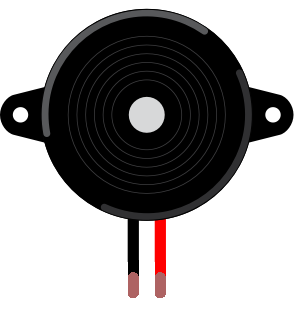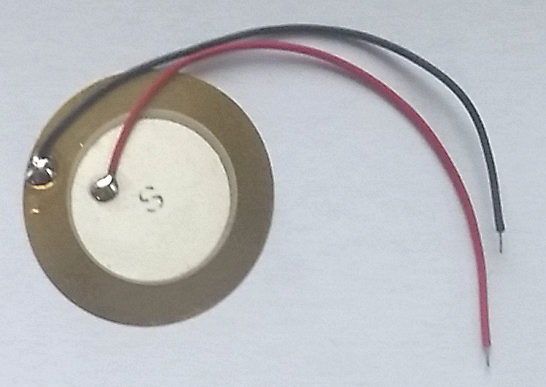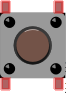# Doorbell

## Introduction

How does Arduino produce sound through a piezo that's connected to one of its digital pins?

## Parts

Do this

Collect these parts and get ready to make the next circuit!

In addition to these parts, you will need an Arduino UNO or Mehackit Board, a USB cable, a breadboard and a bunch of jumper wires.

PartImageDescription
Piezo speakerPiezo speakers may look different, here's two examples.

A piezo can transform a quickly alternating voltage into sound. Some piezos can be used as a knock sensor.
You will need a so-called passive piezo for this chapter. Active piezo speakers are a bit more expensive and they are programmed differently (they also work with Arduino!)

ButtonThe button in the Mehackit Maker Kit is white, not brown.

Educator notes

There are two basic types of piezo speakers (or piezo buzzers), active and passive. In this tutorial you’ll use a passive piezo. Active piezos cost a bit more and are programmed differently. Both active and passive piezos work nicely with Arduino, but it is recommended that you use a 100 ohm resistor with an active piezo.

Passive and active piezos may look quite similar! If you have the Mehackit Maker Kit, you’ll have the passive one for sure. Some kits have both, so check out the components listing and search the model number of the piezo if necessary!

An active piezo has built-in components that produce sound immediately when you activate the piezo with a direct current (DC). You can use the `digitalWrite` command to play sounds. However, to control the pitch of the sound you have to manipulate the speed at which the piezo pin is turned on and off.

As you will learn, the tone command is an easy way to make sounds with a passive piezo!

## Circuit

Do this

Make a circuit according to the diagram below.

• Connect the pushbutton to pin 2 and ground (GND)
• Connect the piezo to pin 7 and ground

Here's how you connect a piezo that has pins instead of wires:

Why are there three blue wires in this circuit?

## Programming 1: First Sounds

Do this

Write a new program following the example below.

• Rows that start with " `//` " are comments. You can write the comment first and then your own code after it, like this:
• OR you can leave the comments out.
• make sure you have all the parentheses `( )` and curly brackets `{ }` in place!
• remember – commands must end with a semicolon` ; `
• Check how to write the `tone` command from the video above, or from the Arduino Reference

Code example

EXTRA

• Test what kind of sounds you can create with different pitch and duration values!

``````tone(12, 400, 2000)
``````
What does the command above do?

Educator notes

Example code

``````void setup() {
pinMode(7, OUTPUT);
pinMode(2, INPUT_PULLUP);
}

void loop() {
tone(7, 440, 80);
}
}
``````

## Programming 2: Control Sound with a Variable

Do this

• You can write comments first and then your own code under them, like this:
• OR you can leave the comments out.
• Check the video again if you don't remember how to write the missing commands.

Code example

EXTRA

• Add new `tone` commands with different notes inside the if statements
• Insert a `delay` command between notes so you can hear them! Change the delay values to create rhythms:
• Check out this table of notes if you want to use certain notes! Pick the number after a note name and give it to the tone command as the second argument.

You wrote int in the beginning of your code. What does it mean?
Why do you need to use a variable in this code?

Educator notes

Example code

``````int playSecond = 0;
void setup() {
pinMode(7, OUTPUT);
pinMode(2, INPUT_PULLUP);
}

void loop() {
tone(7, 440, 80); // button down, note 1 is played
playSecond = 1;
} if (digitalRead(2) == HIGH && playSecond == 1) {
tone(7, 250, 200);
delay(800);
tone(7, 230, 100);
delay(1000);
playSecond = 0;
}
}
``````

Alternative way to write the code

There's often more than one way to make an Arduino code work. Here's an other option - you could replace the structure:

`if (digitalRead(2) == HIGH && playSecond == 1)`

with an else if structure:

``````  if (digitalRead(2) == LOW) {
tone(7, 440, 80); // button down, note 1 is played
playSecond = 1;
} else if (playSecond == 1) {
tone(7, 250, 200);
delay(800);
tone(7, 230, 100);
delay(1000);
playSecond = 0;
}
``````

Tone and delay

The students can create rhythms and melodies with the piezo sounds if they want. The result may be something like this:

``````tone(7, 450, 500);
delay(1000);
tone(7, 359, 500);
delay(800);
``````

It is not required to understand just yet how Arduino sets the timing of `tone` and `delay` commands, but the students may wonder how their Arduino rhythms are realized and what the logic is behind the code.

Arduino has built-in timers for keeping track of timed events. These include playing a note, counting how long a delay is or what kind of electric pulses must be fed to a motor.

In the example above, the length of the note is 500ms, and there are delays of 1000ms and 800ms written after each` tone` command. But how long does it take to play through the whole thing?

Instead of first playing a note for 500ms and then having a break of 1000ms, then playing the second note and executing the second delay, Arduino times the events a bit differently:

The first `tone` starts simultaneously with the first `delay` command, and likewise, the second `tone` and second `delay` start at the same time.

This is due to how Arduino's timers work: `tone` uses a different timer than `delay`! But remember, `delay` affects the whole program meaning that no new commands can be executed while a `delay` is "on".

### Conditional statements

In the code you made an if statement, which is very common in programming.

If you have two equal signs (`==`) in the condition of an if statement, it is known as a relational operator - this one means "equals".

Other relational operators are:

• `a <= b`
a is less than or equal to b
• `a < b`
a is less than b
• `a > b`
a is greater than b
• `a >= b`
a is greater than or equal to b
• `a != b`
a and b are not equal

What happens if you make a new variable inside the setup part?

Educator notes

`&&` (double ampersand) is one of the logical (or boolean) operators used in programming. It is also called logical or boolean AND. As described, it is typically used in an if statement with two conditions that are simultaneously true.

In addition to AND, there are other logical operators, as well.

`||` - logical OR

Example:

``````if (digitalRead(2) == LOW || digitalRead(3) == LOW) {
tone(7, 440, 80);
}
``````

Imagine a button connected to both pin 2 and pin 3 - in this case, the note defined in the `tone` function will be played when either of the buttons is pressed

`!` - logical NOT

Example:

``````if ( !(digitalRead(2) == LOW)) {
tone(7, 440, 80);
}
``````

Now the tone is played if the button in pin 2 does not produce the reading LOW. This particular example could be written differently - it is the same as:

``````if ( digitalRead(2) == HIGH) {
tone(7, 440, 80);
}
``````

Logical NOT is a quick and practical way to invert a program's logic in many cases.